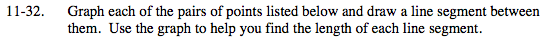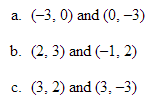### Home > MC2 > Chapter 11 > Lesson 11.2.1 > Problem11-32

11-32.Draw a triangle using the segment as your hypotenuse. Then find out the lengths of the legs.

Use the Pythagorean Theorem to find the length of the hypotenuse.

32 + 32 = hypotenuse2
Now solve for the hypotenuse.

$\sqrt{18} \approx 4.24 \;\text{units}$

See part (a).

Use the Pythagrean Theorem to find the length of the segment.

Use the graph to find the length of the segment.

Use the eTool below to graph each segment.
Click the link at right for the full version of the eTool: MC2 11-32 HW eTool